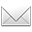# 头脑中没有作品，好比飞机没有航向。············* * *

The theorem above generalizes to the following result.

---- 定理1.1推广为如下结果.

.

Theorem 1.3. The absolute Galois groups of K and K are canonically isomorphic.

---- 简记 {K} {K}.

---- 群的元素都是映射. 若由集合产生出群，则必然先产生(可逆)映射.

.

Our aim is to generalize this to a comparison of geometric objects over K with geometric objects over K.

---- 目的是将此推广，用于比较两个完域上的几何对象.

.

The basic claim is the following.

---- 基本结果如下.

---- (待续).

.

*

1. 完域：ndv(1)~K~(Φ)=K/p.

---- 完全拓扑域带两个约束.

---- 其一，指定某种拓扑；其二，引入某类映射.

.

2. K(p) 关联到 K，其可乘幺半群为 K = lim<K (x x^p).

---- 方法：Fontaine 构造.

.

---- K°/p  K°/p.(para.3a)

---- K = lim<K, x x^p.(para.3b)

----  (x)d --> (x#)d

........分裂域

[K] ~>  [K]c

http://blog.sciencenet.cn/blog-315774-1174703.html

## 全部精选博文导读

GMT+8, 2019-5-20 09:17

Powered by ScienceNet.cn

Copyright © 2007- 中国科学报社